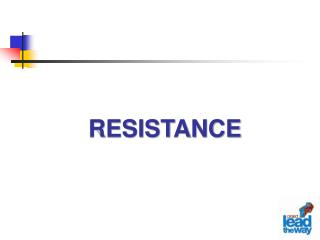DownloadDownload PresentationRESISTANCE

# RESISTANCE

Télécharger la présentation## RESISTANCE

- - - - - - - - - - - - - - - - - - - - - - - - - - - E N D - - - - - - - - - - - - - - - - - - - - - - - - - - -
##### Presentation Transcript

1. RESISTANCE

2. DEFINED - Opposition to electron flow. - The resistance in a metal conductor is directly proportional to the length and inversely proportional to the cross-sectional area. R=Resistance р=specific resistance l=length A=Cross-Sectional Area

3. DEFINED - Increase in temperature will also increase resistance. -Resistance is measured in Ohms (Ω) -Resistance sounds like a bad thing but we tend to use it to our advantage in most circuits.

4. Current Current Resistance THEORY Thus anytime current needs to be limited in a circuit, a resistor can be added. Notice as the resistance increases the current decreases.

5. Schematics Fixed Resistors Potentiometers RESISTORS Resistors are devices that turn electrical current into heat energy. This is valuable to limit current flow to another portion of a circuit. There are two main types of resistors, fixed and variable. The variable resistor may also be called a potentiometer. The schematic symbols for each type are on the right. The arrow is used to denote a resistor that is variable or controllable. How can we determine the resister’s value? There are two ways - measuring with a meter or reading the color code.

6. Resistance Color Code First Number Second Number Tolerance /Accuracy Fixed resistors use color bands to make it easy to read their resistance from any angle or position Number of Zeros or if: Gold - divide by 10 Silver - divide by 100

7. Number of Zeros Accuracy Second Number First Number Black = 0 Brown = 1 Red = 2 Orange =3 Yellow = 4 Green =5 Blue = 6 Violet = 7 Gray = 8 White = 9 Third color band: gold= divide by 10 silver = divide by 100 Fourth color band: gold = 5 % = .05 silver = 10 % = .10 4 7 0 0 Ohms @ 10 %accuracy

8. Highest Lowest Black = 0 Brown = 1 Red = 2 Orange =3 Yellow = 4 Green =5 Blue = 6 Violet = 7 Gray = 8 White = 9 Third color band: gold= divide by 10 silver = divide by 100 Fourth color band: gold = 5 % = .05 silver = 10 % = .10 Use the accuracy band to determine the resistors acceptable range of resistance 5 6 0 0 0 @ 10 % 5 6 0 0 0 Acceptable Range .10 5 6 0 0 0 5 6 0 0 0 5 6 0 0.0 0 + 5 6 0 0 - 5 6 0 0 6 1 6 0 0 5 0 4 0 0